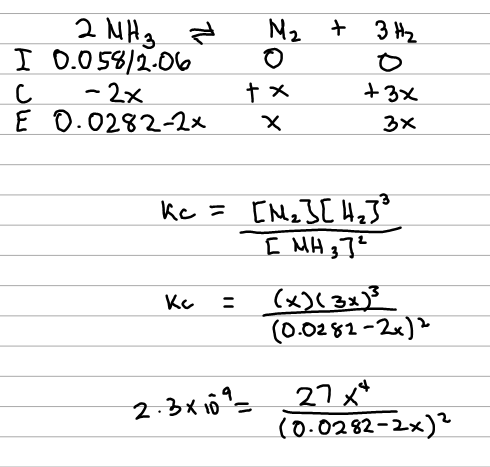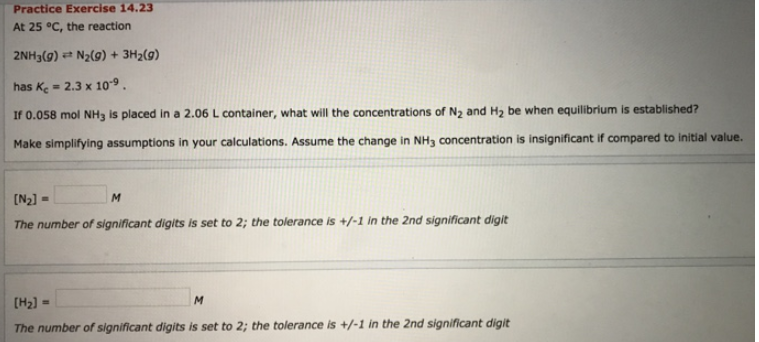# Problem: At 25°C, the reaction 2NH3(g) ⇌ N2(g) + 3H2(g) has Kc = 2.3 x 10-9. If 0.058 mol NH3 is placed in a 2.06 L container, what will the concentrations of N2 and H2 be when equilibrium is established? Make simplifying assumptions in your calculations. Assume the change in NH3 concentration is insignificant if compared to initial value.

###### FREE Expert Solution83% (453 ratings)###### Problem Details

At 25°C, the reaction

2NH3(g) ⇌ N2(g) + 3H2(g)

has Kc = 2.3 x 10-9. If 0.058 mol NH3 is placed in a 2.06 L container, what will the concentrations of N2 and H2 be when equilibrium is established?

Make simplifying assumptions in your calculations. Assume the change in NH3 concentration is insignificant if compared to initial value.